# Length - 6th grade (11y) - math problems

#### Number of problems found: 207

• GrandmaWe're going to Grandma. We still have 24 km left. How far does Grandma live if we have traveled from the total distance: half, a third, a fifth?
• Tractor wheelsThe front wheel of the tractor has a circumference of 18 dm and the rear 60 dm. We will make a red mark on the lowest point of both wheels. The tractor then starts. At what distance from the start will both marks appear identically at the bottom again?
• PegsFrom two sticks 240 cm and 210 cm long, it is necessary to cut the longest possible pegs for flowers so that no residues remain. How many pegs will it be?
• JagdeepJagdeep had a strip of paper measuring 1.8 m. He cut it into 4 equal strips. How long is each shorter strip of paper?
• SurianiSuriani had 3.05 m of ribbon. She used up 0.76 m of it. What is the length of ribbon that she has left?
• Isosceles trapezoidFind the area of an isosceles trapezoid with bases of 8cm and 72mm. The height of the trapezoid is equal to three-quarters of the longer base.
• Two ribbonsThe total length of the two ribbons is 13 meters. If one ribbon is 7 and 5/8 meters long, what is the length of the other ribbon?
• Cyclist 12What is the average speed of a cycle traveling at 20 km in 60 minutes in km/h?
• My fatherMy father cut 78 slats on the fence. The shortest of them was 97 cm long, the longer one was 102 cm long. What was the total length of the slats in cm?
• Identical cubesFrom the smallest number of identical cubes whose edge length is expressed by a natural number, can we build a block with dimensions 12dm x 16dm x 20dm?
• Winch drum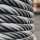Originally an empty winch drum with a diameter of 20 cm and a width of 30 cm on the rescue car, he started winding a rope with a thickness of 1 cm beautifully from edge to edge. The winch stopped after 80 turns. It remains to spin 3.54m of rope (without h
• The swallow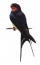The swallow will fly 2.8 km per minute. How many km will the swallow fly in one hour?
• The tourist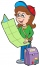The tourist has walked 3/4 of the route. The destination is 12.5 km away. How many kilometers did the route measure?
• Doug bikedDoug biked 5 1/4 miles in 3/4 of an hour. What is his average speed?
• TableclothsThe restaurant has sixty-two square tablecloths with a side length of 150 cm and 36 rectangular tablecloths with dimensions of 140 cm and 160 cm. A) How many meters of hemming ribbon will be needed if we add 50 cm to each tablecloth? B) The ribbon sale in
• Apartment areaAnton wanted to measure the area of the apartment. But he bought a meter only 1.5m long. Later he recalled that he had two meters long at 4.5m and 18m at home. How many times are home gauges longer than the meter he bought?
• Two villages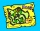On the map with a scale of 1:40000 are drawn two villages actually 16 km away. What is their distance on the map?Calculate the radius of the circle whose length is 107 cm larger than its diameter
• Cottage house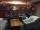The village is 28 km from the cottage. Father goes from village to cottage. Son goes from the cottage to the village. They meet 10 km further behind the cottage. How much did dad walk?
• The perimeter 3The perimeter of a rectangle is 35 cm. The ratio of the length to its width is 3:2. Calculate the dimensions of the rectangle

Do you have an interesting mathematical word problem that you can't solve it? Submit a math problem, and we can try to solve it.

We will send a solution to your e-mail address. Solved examples are also published here. Please enter the e-mail correctly and check whether you don't have a full mailbox.

Please do not submit problems from current active competitions such as Mathematical Olympiad, correspondence seminars etc...

Do you want to convert length units?